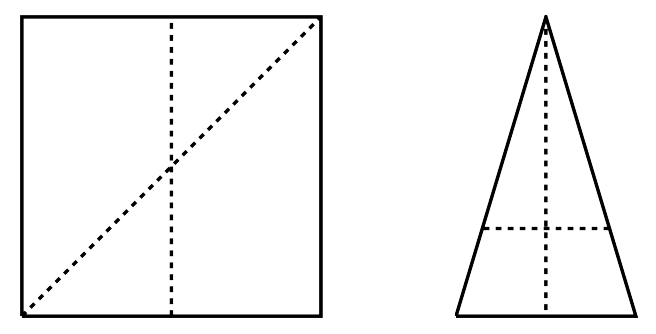시간 제한 메모리 제한 제출 정답 맞은 사람 정답 비율
2 초 512 MB 19 4 4 26.667%

문제

You are given a flat piece of chocolate of convex polygon shape. You are to cut it into two pieces of precisely the same amount with a straight knife.

Write a program that computes, for a given convex polygon, the maximum and minimum lengths of the line segments that divide the polygon into two equal areas.

The figures below correspond to first two sample inputs. Two dashed lines in each of them correspond to the equal-area cuts of minimum and maximum lengths.Figure F.1. Sample Chocolate Pieces and Cut Lines

입력

The input consists of a single test case of the following format.

n
x1 y1
.
.
.
xn yn

The first line has an integer n, which is the number of vertices of the given polygon. Here, n is between 3 and 5000, inclusive. Each of the following n lines has two integers xi and yi, which give the coordinates (xi, yi) of the i-th vertex of the polygon, in counterclockwise order. Both xi and yi are between 0 and 100 000, inclusive.

The polygon is guaranteed to be simple and convex. In other words, no two edges of the polygon intersect each other and interior angles at all of its vertices are less than 180.

출력

Two lines should be output. The first line should have the minimum length of a straight line segment that partitions the polygon into two parts of the equal area. The second line should have the maximum length of such a line segment. The answer will be considered as correct if the values output have an absolute or relative error less than 10−6.

예제 입력 1

4
0 0
10 0
10 10
0 10


예제 출력 1

10
14.142135623730950488


예제 입력 2

3
0 0
6 0
3 10


예제 출력 2

4.2426406871192851464
10.0


예제 입력 3

5
0 0
99999 20000
100000 70000
33344 63344
1 50000


예제 출력 3

54475.580091580027976
120182.57592539864775


예제 입력 4

6
100 350
101 349
6400 3440
6400 3441
1200 7250
1199 7249


예제 출력 4

4559.2050019027964982
6216.7174287968524227